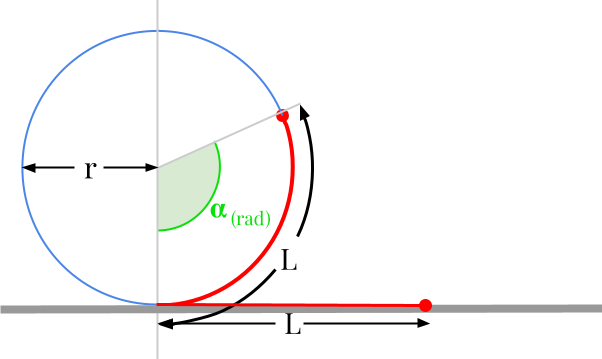Question #6145

Merged questions

You can't actually convert meters to radians. Radian is a measure of angle, while millimeters is a unit of distance. There is an homogeneity issue between the two quantities.However, there are many mechanical systems that transform linear motion into rotary motion. The most obvious system is the wheel: the rotation of the wheel moves the vehicle forward and vice versa. In this case, the formula governing such a system and allowing to transform a displacement given in millimeters into a rotation of an angle expressed in radians is given by:

﻿﻿

﻿﻿

with :

• ﻿﻿ the distance or length expressed in millimeters [mm]
• ﻿﻿ is the radius expressed in meters [m]